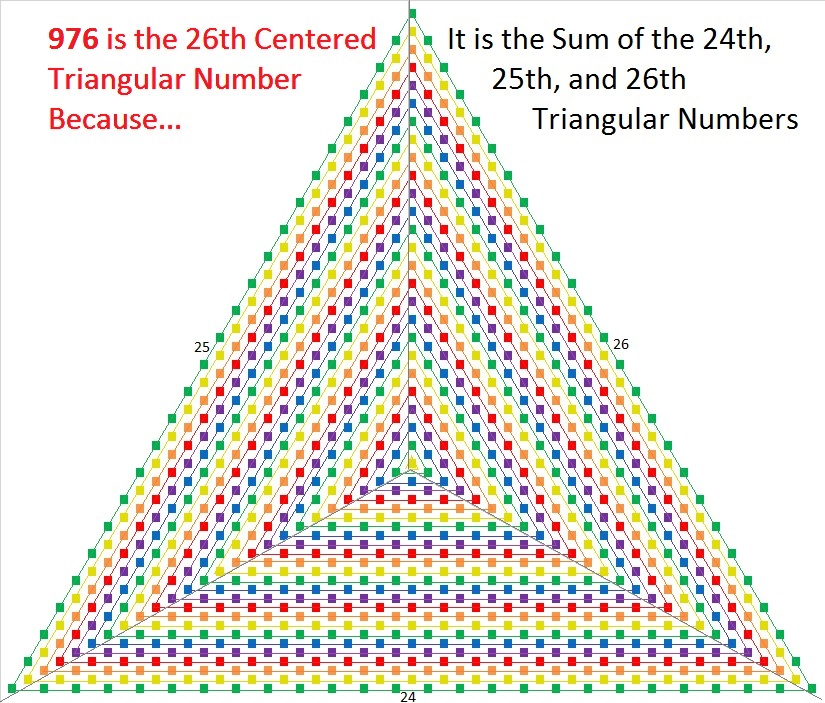# What Kind of Shape is 976 in?

If you look at a list of Centered Triangular Numbers, 976 will be the 26th number on the list. 976 is the sum of the 24th, 25th, and 26th triangular numbers. That means
(24×25 + 25×26 + 26×27)/2 = 976

There’s a formula to compute centered triangular numbers, and this one is found by using
(3(25²) + 3(25) + 2)/2 = 976

As far as the formula is concerned, it is the 25th centered triangular number even though it is the 26th number on the list. I’m calling it the 26th centered triangular number because counting numbers on a list is easier than using a formula.976 is also the 16th decagonal number because 4(16²) – 3(16) = 976. I couldn’t resist illustrating that 10-sided figure.16 × 61 is a palindromic expression that happens to equal 976.

976 is also a palindrome when written in some other bases:
It’s 1100011 in BASE 3 because 3⁶ + 3⁵ + 3¹ + 3⁰ = 976,
808 in BASE 11, because 8(11²) + 0(11¹) + 8(11⁰) = 976
1E1 in BASE 25 (E is 14 base 10) because 1(25²) + 14(25¹) + 1(25⁰) = 976

24² + 20² = 976 That makes 976 the hypotenuse of a Pythagorean triple:
176-960-976 calculated from 24² – 20², 2(24)(20), 24² + 20²

• 976 is a composite number.
• Prime factorization: 976 = 2 × 2 × 2 × 2 × 61, which can be written 976 = 2⁴ × 61
• The exponents in the prime factorization are 4 and 1. Adding one to each and multiplying we get (4 + 1)(1 + 1) = 5 × 2 = 10. Therefore 976 has exactly 10 factors.
• Factors of 976: 1, 2, 4, 8, 16, 61, 122, 244, 488, 976
• Factor pairs: 976 = 1 × 976, 2 × 488, 4 × 244, 8 × 122, or 16 × 61
• Taking the factor pair with the largest square number factor, we get √976 = (√16)(√61) = 4√61 ≈ 31.240999This site uses Akismet to reduce spam. Learn how your comment data is processed.